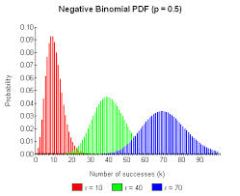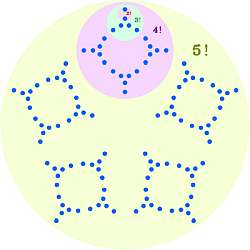# Binomial Probability Calculator

Instructions: Use our Binomial Probability Calculator with steps to compute binomial probabilities using the form below. Please type the population proportion of success p, and the sample size n, and provide details about the event you want to compute the probability for :Population proportion of success (p) =Sample Size (n) =
Two-Tailed:
≤ X ≤
Left-Tailed:
X ≤
Right-Tailed:
X ≥

## Binomial Probability Calculator

More about the binomial distribution probability so you can better use this binomial calculator: The binomial probability is a type of discrete probability distribution that can take random values on the range of $$[0, n]$$, where $$n$$ is the sample size.

### Properties of the Binomial Probability

The main properties of the binomial distribution are:

• It is discrete, and it can take values from 0 to n, where n is the sample size

• The type of skewness depends on the parameters n and p

• It is determined by two parameters: the population proportion of success p, the sample size n (or number of trials)

• The mean of the binomial distribution is $$n\cdot p$$ and its standard deviation is $$\sqrt{np(1-p)}$$

### What is the Binomial Probability Formula?

The formula that defines the binomial probability (which is called its probability distribution function ) is:

$\Pr(X = k) = \left( \begin{matrix} n \\\\ k \end{matrix} p^k \cdot (1-p)^{n-k}$

where n and p are the corresponding parameters of the distribution.### How to use this binomial distribution calculator with steps

Using the above binomial distribution curve calculator, we are able to compute probabilities of the form $$Pr(a \le X \le b)$$, of the form $$\Pr(X \le b)$$ or of the form $$\Pr(X \ge a)$$.

Type the appropriate parameters for $$n$$ and $$p$$ in the text box above, select the type of tails, specify your event and compute your binomial probability, showing all step-by-step details of the binomial probability formula.

### Other important probability distribution calculators

The binomial distribution is a kind of discrete distribution. Other calculators available for discrete distributions are our Poisson distribution calculator, hyper-geometric calculator or our geometric distribution calculator.

### What happens when the probability of success is not constant?

A generalized form of the binomial coefficient is the multinomial coefficient, which considers combinations of $$k$$ numbers that add up to $$n$$, with $$k \ge 2$$.

Now, if you are dealing with continuous distribution, you may like to check out our normal probability calculator online, which deals with the normal distribution and related events, which is the most common continuous distribution.### Example: Calculation of Binomial Probabilities

Question: Assume that X is a random variable with a binomial distribution, with parameters n = 10 and p = 0.45. Compute $$\Pr(2\le X\le 4)$$.

Solution:

We need to compute a binomial distribution probability. The following information are provided:

 Population Probability of Success $$(p)$$ = $$0.45$$ Sample Size $$(n)$$ = $$10$$ Probability Event = $$\Pr(2 \leq X \leq 4)$$

This implies that

$\Pr(2 \le X \le 4) = \Pr(X = 2) + \Pr(X = 3) + \Pr(X = 4)$$= \left( \begin{matrix} 10 \\\\ 2 \end{matrix}\right) 0.45^{ 2} \cdot 0.55^{ 10-2} + \left( \begin{matrix} 10 \\\\ 3 \end{matrix}\right) 0.45^{ 3} \cdot 0.55^{ 10-3} + \left( \begin{matrix} 10 \\\\ 4 \end{matrix}\right) 0.45^{ 4} \cdot 0.55^{ 10-4}$$= 0.0763 + 0.1665 + 0.2384$ $= 0.4811$

which means that the probability we are looking for is $$\Pr(2 \leq X \leq 4) = 0.4811$$.